# Binocular visual positioning under inhomogeneous, transforming and fluctuating media

Binocular visual positioning under inhomogeneous, transforming and fluctuating media

Song Wang | Yanzhu Hu

Automation College, Beijing University of Posts and Telecommunications, Beijing 100876, China

Corresponding Author Email:
yzhu@263.net
Page:
253-276
|
DOI:
https://doi.org/10.3166/TS.35.253-276
|
Accepted:
|
Published:
31 December 2018
| Citation

OPEN ACCESS

Abstract:

This paper aims to disclose the effects of abnormal media (i.e. inhomogeneous media, transforming media and fluctuating media) on binocular vision positioning. For this purpose, a new model of binocular visual positioning was established to solve the media transforming and inhomogeneity. Then, the cloud model was introduced to modify the Kalman filter to tackle the positioning uncertainty caused by media fluctuation. Experimental results show that the proposed approach can realize accurate binocular visual positioning in inhomogeneous, transforming and fluctuating media. The research findings may promote the application of vision positioning technology.

Keywords:

inhomogeneous media, transforming media, media fluctuation, binocular visual positioning, uncertainty, kalman filter, cloud model

1. Introduction

Visual positioning, the key technology to provide information on target position (Henten et al., 2003), has been adopted for various purposes, such as the self-picking in agriculture (Xiong et al., 2012; Yin et al., 2012; Wang et al., 2015), the self-machining and self-testing in industry (Prado et al., 2015; Mahendran and Philip, 2012), the visual positioning in service industry (Lang et al., 2014; Ding et al., 2016) and the self-locating in military (Chen and Guo, 2016). In essence, visual position is a machine vision technique mimicking the operations of human eyes.

Much research has been done on the application of visual positioning in VO system (He et al., 2013), 3D reconstruction (Alexiadis et al., 2013), self-driving (Kexin, 2015), pollution reduction (Michaels et al., 2013), hand-eye system (Hu and Li, 2016) and so on. However, these studies on visual positioning are confined to a single, homogenous and static medium, failing to tackle the complex real-world conditions like underwater fishing (Chen et al., 2011) and submarine reconnaissance (Wang et al., 2012). Thus, the research results cannot be directly applied in the inhomogeneous and dynamic media. Otherwise, the positioning data will contain lots of errors, and the simulated positions will become untrue points. The uncertainty of visual positioning in complex media has attracted much attention in the academic field, yielding fruitful but preliminary results.

Some scholars explored the visual positioning under the condition of multiple transforming media. For instance, Bian et al. (2016) developed an accurate underwater visual positioning system for light beams. Kohut et al. (2016) designed an underwater robot with visual positioning ability for reservoir maintenance. Wang et al. (2010; 2011) were the first to discuss the visual positioning in two transforming media and three transforming media. Wang (2011) studied the binocular vision positioning in multiple transforming media. During the research, the Kalman filter and its modified versions (e.g. extended Kalman filter and adaptive Kalman filter) are often adopted to eliminate the noises of medium fluctuation (Zhang et al., 2012; Guo, 2008; Zhang et al., 2016).

The above studies were conducted under the assumption of homogenous media and known refractive index of each medium. The condition of inhomogeneous media, under which the refractive index will become dynamic, was not included in these studies, not to mention the condition of inhomogeneous transforming media. In addition, neither was uncertainty taken into account. To make up for these gaps, this paper constructs a model for binocular visual positioning under inhomogeneous transforming media, and combines the cloud model and the Kalman filter into the cloud Kalman approach, aiming to eliminate noises in the modelling process. The research was mainly carried out in the following steps.

Firstly, a physical model with two optical paths was established for binocular visual positioning under transforming media, in which the light is reflected at the interface between each two media, and used to complete the visual positioning of the underwater target. Secondly, the refractive index of each medium, which changes with the purity and homogeneity of the medium, was solved by the optical path equations in the model. Thirdly, the cloud model was employed to adjust the control parameters of the Kalman filter, suppress the noises from the random fluctuations of the media, and analyze the 3D information of the target point. Finally, the cloud-Kalman method was verified through experiments with three uncertain factors. The experimental results show that our approach can outperform other visual positioning strategies.

2. Binocular vision positioning technologies

There are three popular visual positioning technologies, namely, the RGB-D (Oliver et al., 2016; Pala et al., 2016), the monocular visual positioning (Zhu and Qiang, 2001) and the binocular visual positioning (Hou et al., 2012). The RGB-D was adopted for Mycroft’s Kinect for Xbox One. This technology can extract depth information directly, but at a high cost and poor accuracy. Relying on fixed changes of camera positions, the monocular visual positioning technology is unsteady due to the periodic motion of camera. Compared with these techniques, the binocular visual positioning can locate the target stably and conveniently based on the relationship between the positions and images of two cameras. This technology can also offset to the position deviation resulted from different media. In light of the above, a binocular visual positioning model was set up for our research (Figure 1).

## 1.jpgFigure 1. Binocular visual positioning model.

The visual positioning is realized through the transformation between three coordinate systems, the world coordinate system, the camera coordinate system and the image coordinate system. According to Zhang’s method, the transformation from image to camera coordinate systems usually precedes that from camera to world coordinate systems. Based on the differences between the two cameras in image coordinate systems, it is possible to obtain the 3D information in the world coordinate system, laying the basis for accurate visual positioning.

2.1. Determining the distortion coefficient in the image coordinate system

Owing to manufacturing limitations, the light path might not pass through the center of camera lens. This error is known as the distortion. Here, the Brown model (Fraser, 1997) is introduced to determine the distortion coefficient of each camera. For the image coordinate system, suppose $k_1$ and $k_2$ are the radial distortion coefficients, $k_3 and$k_4$are the tangential distortion coefficients,$\left(u_{0}, v_{0}\right)$and$\left(u_{1}, v_{1}\right)$are the coordinates of the image and the target, respectively, and$\left(u_{0}^{\prime}, v_{0}^{\prime}\right)$and$\left(u_{1}^{\prime}, v_{1}^{\prime}\right)$are the coordinates of the image and the target, respectively, after distortion calibration. Then, the results of distortion calibration can be expressed as:$\begin{aligned} \Delta u=& k_{1} \cdot \Delta u^{\prime} \cdot r^{2}+k_{2} \cdot \Delta u^{\prime} \cdot r^{4} \\ &+2 k_{3} \cdot \Delta u^{\prime} \cdot \Delta v^{\prime}+k_{4} \cdot\left(r^{2}+2 \cdot \Delta u^{\prime 2}\right) \end{aligned}$(1)$\begin{aligned} \Delta v=& k_{1} \cdot \Delta v^{\prime} \cdot r^{2}+k_{2} \cdot \Delta v^{\prime} \cdot r^{4} \\ &+2 k_{3} \cdot \Delta u^{\prime} \cdot \Delta v^{\prime}+k_{4} \cdot\left(r^{2}+2 \cdot \Delta v^{\prime 2}\right) \end{aligned}$(2) where$r^{2}=\Delta u^{\prime 2}+\Delta v^{\prime 2} ; \Delta u=u_{1}-u_{0} ; \Delta v=v_{1}-v_{0} ; \Delta u^{\prime}=u_{1}^{\prime}-u_{0} ; \Delta v^{\prime}=v_{1}^{\prime}-v_{0}^{\prime}$. 2.2. Transformation from the image coordinate system to camera coordinate system After lens calibration, the pixel information in the image coordinate system was transformed to size information in the camera coordinate system. The transformation matrix, hereinafter referred to as the internal matrix, was obtained by Zhang’s method to derive the coordinates of the target in the camera coordinate system:$\left[\begin{array}{lll}{p} & {q} & {1}\end{array}\right]^{\mathrm{T}}=K_{\text {in}}^{-1} *\left[\begin{array}{lll}{u^{\prime}} & {v^{\prime}} & {1}\end{array}\right]^{\mathrm{T}}$(3) where$K_{i n}=\left[\begin{array}{lll}{f_{u}} & {c} & {u_{0}^{\prime}} \\ {0} & {f_{v}} & {v_{0}^{\prime}} \\ {0} & {0} & {1}\end{array}\right]$, with$f_u$and$f_v$being the ratios of image pixels to image size in x axis and$y$axis direction, respectively. 2.3. Relative positions between the two cameras in the world coordinate system The transformation matrices between the two cameras in the binocular visual positioning technology, hereinafter referred to as the external matrix$K_{o u t}$can be expressed as:$K_{o u t}=\left[\begin{array}{ll}{R} & {t} \\ {0} & {1}\end{array}\right]$(4) where R is the rotation matrix; t is the displacement matrix. With one camera at the original position and the other at the terminal position, the rotation matrix was formed by rotating the system about the three axes, while the displacement matrix was obtained according to the relative displacement along each axis. Hence, the relationship between the two cameras in the world coordinate system can be described as:$\left[\begin{array}{llll}{x} & {y} & {z} & {1}\end{array}\right]_{l e f t}^{\mathrm{T}}=K_{o u t} \cdot\left[\begin{array}{llll}{x} & {y} & {z} & {1]_{r i g h t}^{\mathrm{T}}}\end{array}\right.$(5) where$\left[\begin{array}{lll}{x} & {y} & {z}\end{array}\right]_{\text {left}}^{T}$and$\left[\begin{array}{lll}{x} & {y} & {z}\end{array}\right]_{r i g h t}^{T}$are the coordinates of the left and right cameras in the world coordinate system, respectively. 2.4. Transformation from the camera coordinate system to the world coordinate system The distance of the target in the world coordinate system was worked out in light of the real size in the coordinate systems of the left and right cameras, making it possible to determine the ratio of the image coordinate system to the world coordinate system. In the world coordinate system, the origin is the position of the left camera, the x axis is parallel to the horizontal plane, the y axis is vertical to the horizontal plane, the z axis is vertical to the line between the two cameras. Then, the ratio of the image coordinate system to the world coordinate system can be determined as:$l=\frac{\|t\|_{2}}{\left\|[p \quad q \quad 1]_{l e f t}^{T}-R \cdot[p \quad q \quad 1]_{r i g h t}^{T}\right\|_{2}}=\frac{d}{f}$(6) The 3D coordinates of the target can be expressed as:$\left[\begin{array}{lll}{x} & {y} & {z}\end{array}\right]=\frac{1}{2}\left(\left[\begin{array}{lll}{x} & {y} & {z}\end{array}\right]_{\text {left}}+\left[\begin{array}{lll}{x} & {y} & {z}\end{array}\right]_{\text {right}}\right)$(7) where$\left[\begin{array}{lll}{x} & {y} & {z}\end{array}\right]_{\text {left}}^{T}=l \cdot\left[\begin{array}{lll}{p} & {q} & {f]_{\text {left}}^{T}}\end{array}\right.$and$\left[\begin{array}{llll}{x} & {y} & {z}\end{array}\right]_{\text {right}}^{T}=l \cdot\left[\begin{array}{lll}{p} & {q} & {f]_{\text {right}}^{T}}\end{array}\right.$are the positions of the left and right cameras, respectively. 2.5. Determining the feature points of the target In this paper, red is used to highlight the target in the color image. The feature points of the target were determined as follows. Step 1: Each pixel has an RGB value in the color image. The target was selected from the image by pinpointing the pixels with the RGB value of the red color. Step 2. The positions of the target pixels were determined one by one. Step 3. After confirming the range of pixel positions, the four extreme boundary values (i.e. the upper one, the lower one, the left one and the right one) were determined for the range. Step 4. The target center position was derived from the four extreme boundary values. 3. Visual positioning under inhomogeneous transforming media As shown in Figure 2, the coordinate system of our visual positioning system has its origin at the terminal of the optical axis of the right camera. In this system, the y axis is vertical to the horizontal plane with the upward direction as the positive direction; the z axis is parallel to the horizontal plane and vertical to the right camera lens, with the forward direction as the positive direction; the x axis is vertical to both the y axis and the z axis, with the left camera in the positive direction. ## 2.jpg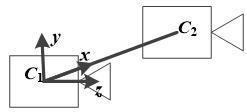Figure 2. The coordinate system of our visual positioning system. 3.1. Visual positioning under transforming media During visual positioning, the light beam travels from the target to the cameras via two media. As the two media differ in refractive indices, the light beam will be refracted at the interface of the two media. Here, the cameras are placed in the air while the target is located underwater. Assuming that each medium is homogenous, the author created a mathematical model for visual positioning (Figure 3). ## 3.jpg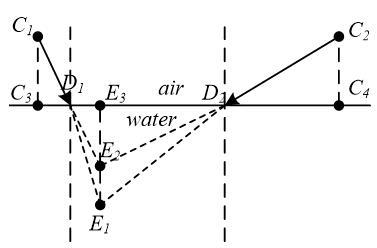Figure 3. The visual positioning model under transforming media. In Figure$3, C_{1}$and$C_{2}$are the positions of the two cameras;$D_{1} D_{2}$is the plane between the two media;$C_{3}$and$C_{4}$are projections of$C_{1}$and$C_{2}$in plane$D_{1} D_{2}$, respectively;$E_{1}, E_{2}$and$E_{3}$are the actual position, the virtual position and the projection in plane$D_{1} D_{2}$of the target, respectively. The refractive index of the air is$n_{1}=1$and that of water is$n_{2}=1.33$. According to the refractive index model of optical physics, the refractive index can be calculated as: refractive index$=\frac{\text { sine of the incident angle }}{\text { sine of the exit angle }}$(8) The following equation can be derived from the model in Figure 1 and equation (8):$\begin{aligned} \frac{n_{1}}{n_{2}} &=\frac{\sin \left(0.5 \pi-\angle C_{1} D_{1} C_{3}\right)}{\sin \left(0.5 \pi-\angle E_{1} D_{1} E_{3}\right)} \\ &=\frac{\cos \angle C_{1} D_{1} C_{3}}{\cos \angle E_{1} D_{1} E_{3}}=\frac{\left|C_{3} D_{1}\right|}{\left|C_{1} D_{1}\right|} \cdot \frac{\left|E_{1} D_{1}\right|}{\left|D_{1} E_{3}\right|} \end{aligned}$(9) In binocular visual positioning, the coordinates of some key points were configured as$C_{1}=(0 \quad 0 \quad 0)$and$E_{2}=\left(x_{2} \quad y_{2} \quad z_{2}\right) .$Assuming that material plane satisfies$y=m,$we have$C_{1}=(0 \quad m \quad 0), E_{3}=\left(x_{2} \quad m+3 \quad z_{2}\right)$and$E_{1}=\left(x_{2} \quad X \quad z_{2}\right) .$The above coordinates are the projections of the two cameras in the plane between the two different media. According to the equations for$C_{1} E_{2}$and$D_{1} D_{2},$the coordinates of point$D_{1}$in the world coordinate system can be derived as:$D_{1}=\left[\begin{array}{lll}{x_{4}} & {y_{4}} & {z_{4}}\end{array}\right]^{\mathrm{T}}=\left[\begin{array}{ccc}{m \cdot \frac{x_{2}}{y_{2}}} & {m} & {m \cdot \frac{z_{2}}{y_{2}}}\end{array}\right]^{\mathrm{T}}$(10) Then, equation (9) can be simplified as:$\frac{n_{1}}{n_{2}}=\frac{\left|C_{3} D_{1}\right|}{\left|C_{1} D_{1}\right|} \cdot \frac{\left|E_{1} D_{1}\right|}{\left|D_{1} E_{3}\right|}=\frac{\left|E_{3} D_{1}\right|}{\left|E_{2} D_{1}\right|} \cdot \frac{\left|E_{1} D_{1}\right|}{\left|D_{1} E_{3}\right|}=\frac{\left|E_{1} D_{1}\right|}{\left|E_{2} D_{1}\right|}$(11) Equation (11) can be rewritten as:$\left(n_{1}^{2}-n_{2}^{2}\right)\left(x_{2}-m \frac{x_{2}}{y_{2}}\right)^{2}+n_{1}^{2}\left(y_{2}-m\right)^{2}-n_{2}^{2}(X-m)^{2}+\left(n_{1}^{2}-n_{2}^{2}\right)\left(z_{2}-m \frac{z_{2}}{y_{2}}\right)^{2}=0$(12) 3.2. Visual positioning under inhomogeneous transforming media The mean refractive index was introduced to solve the light propagation in inhomogeneous media. To simulate the inhomogeneity, salt was added into the water in the above model without stirring. Then, the above model was transformed to a new model in Figure 4, in which the light beam bent along the red curves. ## 4.jpg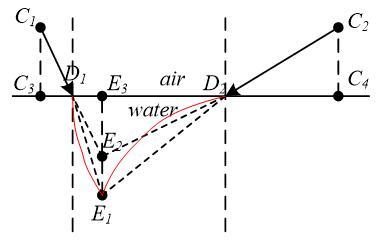Figure 4. The visual positioning model under inhomogeneous transforming media. According to equation (9) and (11), we have:$\frac{n_{1}}{n_{2}}=\frac{\left|E_{1} D_{1}\right|}{\left|E_{2} D_{1}\right|}=\frac{\left|E_{1} D_{2}\right|}{\left|D_{2} E_{2}\right|}$(13) According to the equations for$C_{2} E_{2}$and$D_{1} D_{2},$the coordinates of point$D_{2}$in the world coordinate system can be derived as:$\begin{array}{l}{D_{2}=\left[\begin{array}{ccc}{x_{5}} & {y_{5}} & {z_{5}}\end{array}\right]^{\mathrm{T}}} \\ {=\left[\frac{\left(m-y_{1}\right)\left(x_{2}-x_{1}\right)}{y_{2}-y_{1}}+x_{1}  m\right.} & {\left.\frac{\left(m-y_{1}\right)\left(z_{2}-z_{1}\right)}{y_{2}-y_{1}}+z_{1}\right]^{\mathrm{T}}}\end{array}$(14) Substituting the variable values into equation (13), we have:$\frac{\sqrt{\left(x_{2}-m \frac{x_{2}}{y_{2}}\right)^{2}+(X-m)^{2}+\left(z_{2}-m \frac{z_{2}}{y_{2}}\right)^{2}}}{\sqrt{\left(x_{2}-m \frac{x_{2}}{y_{2}}\right)^{2}+\left(y_{2}-m\right)^{2}+\left(z_{2}-m \frac{z_{2}}{y_{2}}\right)^{2}}}=\frac{\sqrt{\left(x_{2}-\frac{\left(m-y_{1}\right)\left(x_{2}-x_{1}\right)}{y_{2}-y_{1}}-x_{1}\right)^{2}+(X-m)^{2}+\left(z_{2}-\frac{\left(m-y_{1}\right)\left(z_{2}-z_{1}\right)}{y_{2}-y_{1}}-z_{1}\right)^{2}}}{\sqrt{\left(x_{2}-\frac{\left(m-y_{1}\right)\left(x_{2}-x_{1}\right)}{y_{2}-y_{1}}-x_{1}\right)^{2}+\left(y_{2}-m\right)^{2}+\left(z_{2}-\frac{\left(m-y_{1}\right)\left(z_{2}-z_{1}\right)}{y_{2}-y_{1}}-z_{1}\right)}}$(15) In this way, the value of X was obtained. The method makes full use of the two light paths in binocular camera, thereby eliminating the uncertainty in the refractive index caused by the inhomogeneous media. 4. Visual positioning under fluctuation of inhomogeneous transforming media The medium fluctuation may lead to tiny changes of the light path. Since the fluctuation is dynamic and random, it cannot be described by common average methods. Hence, random fluctuation was added to the water in the above model, creating waves on the surface. Then, the deviations of the position information appeared with the waving surface (Figure 5). ## 5.jpgFigure 5. Position deviation resulted from medium fluctuation. Under the above conditions, 100 images were shot successively, leading to the following target positioning results. ## 6.jpgFigure 6. Visual positioning of successive images under medium fluctuation. To eliminate the time-variation in the position information, the Kalman filter was improved by the cloud model such that the position information can converge better to the true values. 4.1. Kalman filter The Kalman filter is an important tool to treat uncertainty problems caused by long-term error (Houtekaer and Zhang, 2016; Houtekamer, 2012). Here, the Kalman filter was improved to solve the uncertainty in position information arising from medium fluctuation. In the world coordinate system, the target position η can be expressed by 3D coordinates x, y and z. The five classical Kalman filter formulas are listed below: (1) State prediction equation:$\eta(k | k-1)=\Psi \cdot \eta(k-1 | k-1)+\Upsilon \cdot U(k)$(16) (2) Covariance prediction equation:$P(k | k-1)=\Psi \cdot \eta(k | k-1) \cdot \Psi^{\mathrm{T}}+Q$(17) (3) Covariance estimation:$\eta(k | k)=\eta(k | k-1)+K g(k) \cdot(\Gamma(k)-H \cdot \eta(k | k-1))$(18) (4) Kalman gain matrix:$K g(k)=P(k | k-1) \cdot H \cdot\left[H \cdot P(k | k-1) \cdot H^{\mathrm{T}}+\mathrm{R}\right]^{-1}$(19) (5) State estimation:$P(k | k)=(E-k g(k) \cdot H) \cdot P(k | k-1)$(20) whereis the number of successive images. The position information of the previous and next images was used in Kalman filter to ensure the convergence under medium fluctuation. 4.2. Multi-dimensional cloud model The control parameters in the state prediction equation of Kalman filter are the key to the optimization of this filter. Many have modified the Kalman filter with control parameters from the artificial neural network (ANN), the adaptive algorithm, etc. (Auger et al., 2013; Li et al., 2012; Xiong et al., 2013) In this paper, the certainty calculated by the cloud model is selected as the control parameter to reduce the negative effect of random fluctuation on Kalman filter, considering the powerful ability of the cloud model to depict uncertainty and randomness [38~40]. Let x(k), y(k), z(k) be the 3D information in each image, with k=1,⋯,n. The randomness of medium fluctuation can be described by the cloud model via parameters like expectation Ex, entropy En and super entropy He. Normally, the cloud model is established through the following steps: (1) Calculate the expectation of samples:$E x(x(k), y(k), z(k))=\frac{1}{k} \sum(x(k), y(k), z(k))$(21) (2) Calculate the entropy of samples:$\operatorname{En}(x(k), y(k), z(k))=\frac{1}{k} \sum\left\{[(x(k), y(k), z(k))-E(x(k), y(k), z(k))]^{2}\right\}$(22) (3) Calculate the super entropy of samples:$H e(x(k), y(k), z(k))=0.1$(23) (4) Generate random numbers$(\hat{x}(k), \widehat{y}(k), \hat{z}(k))$, taking Ex(x(k), y(k), z(k)) and En(x(k), y(k), z(k)) as expectation and standard deviation, respectively. (5) Generate random numbers$n^{\prime}(x(k), y(k), z(k))$, taking En(x(k), y(k), z(k)) and He(x(k), y(k), z(k)) as expectation and standard deviation, respectively. (6) Calculate the certainty:$\mu(k)=\exp \left[-\sum_{x, y, z}^{x, y, z}\left[\frac{(\eta(k)-E x(k))^{2}}{2\left(E n^{\prime}(k)\right)^{2}}\right]\right]$(24) (7) Calculate the certainty of the next image by repeating the above steps, find the smallest certainty by comparing the certainties μ(k) of all images, and take the x(k), y(k), z(k) with the smallest certainty as the target coordinates. 4.3. Superiority of the improved Kalman filter The multi-dimensional cloud model was adopted to improve the Kalman filter, aiming to suppress the effect of fluctuations in inhomogeneous media in binocular visual positioning. The improved Kalman filter has the following advantages. (1) The features of the control parameters in the traditional Kalman filter are fully utilized to control the filtering from the first step. (2) The multi-dimensional cloud model describes the uncertainty clearly. Different from the traditional error, this uncertainty can depict the position information with expectation, variance, entropy and super entropy. (3) The combination between Kalman filter and multi-dimension cloud model enables one-step prediction and gives the uncertainty in each step. As a result, the positioning information of each image is based on the previous image and the sequence of all images, leading to a much better filtering effect. (4) The cloud model only adjusts the control parameters in the state prediction equation of the Kalman filter, rather than affect the entire filtering process. Thus, the adjustment does not increase the time complexity of the Kalman filter. 5. Experimental verification 5.1. Calibration of binocular camera The radial and tangential distortion coefficients of the two cameras (Table 1) were determined by the Brown model. The internal matrices of the two cameras (Table 2) were obtained by Zhang’s calibration method. Table 1. The radial and tangential distortion coefficients of the two cameras.  No. k1 k2 k3 k4 1 -0.307 0.729 0.048 0.013 2 -0.298 0.715 0.049 0.014 Table 2. The internal matrices of the two cameras.  No. fu[mm/px] fv[mm/px] u0[px] v0[px] f[mm] 1 2.97944 2.99419 626.222 494.304 3.1 2 2.97926 2.99325 628.112 486.102 3.1 In the world coordinate system, the coordinates of the two cameras were set to$C_{1}[0,0,0]$and$C_{2}[230,0,0]$, respectively. Meanwhile, the external matrix between the two cameras can be computed as:$K_{o u t}=\left[\begin{array}{ll}{R} & {t} \\ {0} & {1}\end{array}\right]=\left[\begin{array}{cccc}{1.0} & {0.0} & {0.0} & {230.0} \\ {0.0} & {1.0} & {0.0} & {0.0} \\ {0.0} & {0.0} & {1.0} & {0.0} \\ {0} & {0} & {0} & {1}\end{array}\right]$(25) For simplicity, the values of external parameters were rounded to the nearest integer. As shown in Figure 7, a red circular slice was attached to the bottom of a washbowl, with its centroid as the target feature point. ## 7.jpgFigure 7. The target image. The colorful images were screened based on RGB values. The red color was regarded as the target when the R value was greater than 168 and the G value was smaller than 50. The upper, lower, left and right points were attached with great importance in the prototype image (Figure 8). The coordinates of the four points were extracted from the image to determine the target center in Figure 9. ## 8.jpgFigure 8. The prototype image. ## 9.jpgFigure 9. Determination of target center. 5.2. Visual positioning experiments under transforming media. The experimental device was illustrated in Figures 10 and 11. The resolutions of the two cameras were both set to 1,280×960 during the simulation. ## 10.jpg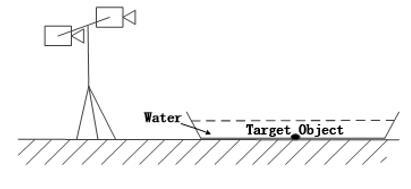Figure 10. Sketch map of the experimental device. ## 11.jpg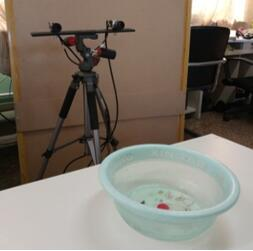Figure 11. An image of the experimental device. One pair of the media images taken by the left and right cameras are given in Figure 12 below. ## 12.jpgFigure 12. Media images taken by the left and right cameras. One pair of the images was selected and the target center coordinates in the image coordinate system were determined (Table 3). Table 3. Target center coordinates in the image coordinate system.  No. Name u[px] v[px] 1 Left camera 560.35 550.69 2 Right camera 840.64 550.52 The data in Tables 2 and 3 were substituted into equation (3), yielding two pairs of target coordinates from left and right cameras: [2.332,-2.912,1] and [1.812,-2.849,1]. Then, the coordinates of E2 were derived as [1023.371,-1277.532,2676.535] from equations (5) and (6). Since m=-800, the coordinates ofwere determined as$E_{1}=[1023.371,-1289.737,2676.535]$. The 3D error between the calculated position and the real position is shown in Figure 13. ## 13.jpg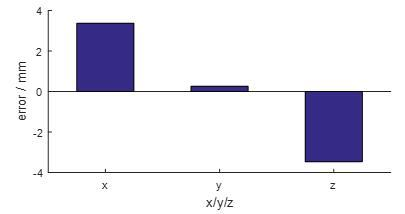Figure 13. 3D error contrast. To verify the positioning accuracy, 100 visual positioning experiments were performed at different target positions. The errors of the three axes were measured in the transforming media and plotted as Figure 14 below. ## 14.jpg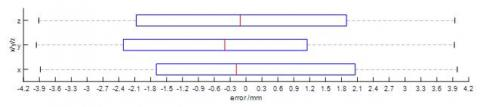Figure 14. 3D error contrast of 100 visual positioning experiments under transforming media. The results show that the errors in the three axes under transforming media were controlled in -4mm and 4mm, indicating that our visual positioning method is feasible and useful. 5.3. Visual positioning experiments under inhomogeneous media To create inhomogeneous media, salt was added to the water in the washbowl without stirring. Usually, a washbowl of water can dissolve 1kg of salt. During the addition, the salt was poured into the same position (Figure 15). Then, the mean refractive index of the inhomogeneous media in our experiments was calculated as 1.33~1.5 according to the refractive index of saturated salt water (1.5~1.6) and that of water (1.33). Following the method in Section 2, the target center coordinates in the image coordinate system were derived (Table 4). ## 15.jpg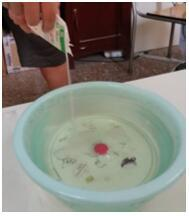Figure 15. Salt addition. Table 4. Target center coordinates in the image coordinate system.  No. Name u[px] v[px] 1 Left Camera 560.67 550.19 2 Right Camera 840.61 550.37 Through the visual positioning of binocular camera, the coordinates of$E_{2}$were confirmed as [1022.997, -1275.321, 2678.759]. Then, the coordinates of$E_{1}$were computed as [1022.997, -1289.737, 2678.759] according to the equation in Section 2.2. In addition, the priori condition n=1.419. To verify the positioning accuracy, 100 visual positioning experiments were performed at different target positions and amounts of salt. The errors of the three axes were measured in the inhomogeneous media and plotted as Figure 16 below. It can be seen from the experimental results that the errors in the three axes all converged to ±0.37mm. Thus, the mean refractive index was calculated by the positioning model. When the priori condition was not n=1.33, the positioning error grew by 12.45%. In this case, the mean refractive index was obtained through the 100 experiments. The calculated values of the mean refractive index all fell between 1.33 and 1.52 (Figure 17), indicating that our method can always locate the target accurately. ## 16.jpg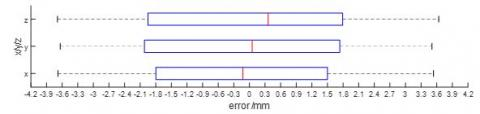Figure 16. 3D error contrast of 100 visual positioning experiments under inhomogeneous transforming media. ## 17.jpg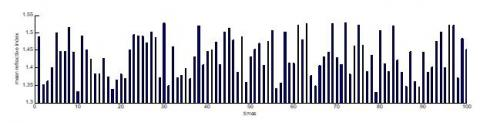Figure 17. The mean refractive index of one hundred experiment. 5.4. Visual positioning experiments under fluctuating media ## 18.jpg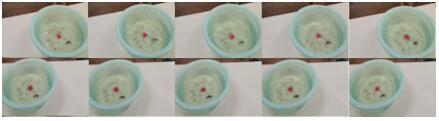Figure 18. Five pairs of successive images taken by left and right cameras. In the previous experiments, the error was controlled well between 3mm and 4mm in three directions. However, the positioning error may increase by 1~2mm under random fluctuations, as shown in the five pairs of images taken successfully under fluctuating media (Figure 18). To eliminate the effect of random fluctuation, 100 images were selected and processed by Kalman filter and the proposed cloud-Kalman filter. The filtered 3D errors are compared with the non-filtered data in Figure 19. ## 19.jpgFigure 19. 3D error contrast between different methods. As shown in Figure 19, the error of the cloud-Kalman method converged to -2.5mm~2.5mm, that of the Kalman filter converged to -3.3mm~3.3mm, and that of the non-filter approach converged to -3.3mm~3.3mm. The results demonstrate the feasibility of the proposed cloud-Kalman algorithm. To verify the positioning accuracy, 100 visual positioning experiments were performed repeatedly. The errors of the three axes were measured under fluctuating media and plotted as Figure 20 below. ## 20.jpgFigure 20. 3D error contrast of 100 visual positioning experiments under fluctuating media. It can be seen from the above figure that the non-filter approach had the largest error range, followed by the Kalman filter, while the cloud-Kalman filter achieved the smallest error range. The error range of the Kalman filter was 10.67% smaller than that of the non-filter approach; the error range of the cloud-Kalman filter was 23.34% smaller than that of the Kalman filter and 31.73% smaller than the non-filter approach. For further validation, the experimental data were processed by the adaptive-Kalman method and backpropagation (BP)-Kalman method. For simplicity, the 3D error ranges were converted to Euclidean distance: error$=\sqrt{\operatorname{error}(x)^{2}+\operatorname{error}(y)^{2}+\operatorname{error}(z)^{2}}\$     (26)

A total of 100 experiments were conducted to compare the performance of the cloud-Kalman, the adaptive Kalman and the BP-Kalman (Table 5).

Table 5. Comparison between the three improved Kalman algorithms.

 No. Contrast index Cloud Kalman Adaptive Kalman BP Kalman 1 The number of experiments with the smallest mean error 97 2 1 2 The range of mean error -4.36mm~4.36mm -4.73mm~4.73mm -4.64mm~4.64mm 3 The processing time per image 5,548.95s 5,672.62s 5,590.16s

The comparison shows that the cloud-Kalman is more suitable for binocular visual positioning under transforming media than the other two improved Kalman filters, thanks to its edge in error range and efficiency.

## 21.jpgFigure 21. Error range-parameter relationship obtained in the 100 experiments.

After that, the parameter impact analysis was carried out based on the data of the 100 experiments. In the proposed cloud-Kalman filter, the uncertainty calculated by the cloud model, falling between zero and one, was adopted as the control parameter of the state prediction equation in the Kalman filter, i.e. the error must be on the order of magnitude of γ. The error convergence range was between -4mm and 4mm. Thus, six parameters were selected to compare their impacts on positioning errors, namely, -4, -3.5, -3, -2.5, -2, -1.5, -1, -0.5, 0.5, 1, 1.5, 2, 2.5, 3, 3.5 and 4. The results are shown in Figure 21 below. The effect of parameter adjustment in the 100 experiments were calculated and recorded in Table 6.

Table 6. Comparison between parameter adjustment effects.

 No. The control parameter The number of experiments with the smallest errors on the x-axis The number of experiments with the smallest errors on the y-axis The number of experiments with the smallest errors on the z-axis 1 -4 0 0 0 2 -3.5 0 0 0 3 -3 0 0 0 4 -2.5 0 0 0 5 -2 0 0 0 6 -1.5 0 0 0 7 -1 0 2 1 8 -0.5 3 2 2 9 0.5 7 3 3 10 1 85 87 91 11 1.5 4 6 2 12 2 1 0 1 13 2.5 0 0 0 14 3 0 0 0 15 3.5 0 0 0 16 4 0 0 0

It can be seen that the control parameter reached an efficiency of over 95% when it was between 0.5 and 1.5, indicating that 0.5~1.5 is the most suitable range for the control parameter of the cloud Kalman method.

6. Conclusions

This paper probes deep to the binocular vision positioning under inhomogeneous media, transforming media and media under fluctuation, and proposes a cloud-Kalman positioning model by improving the Kalman filter with the cloud model. Through repeated experiments, it is proved that the proposed cloud-Kalman positioning model can effectively solve the binocular visual positioning problem under transforming media or inhomogeneous transforming media, and eliminate the uncertainty caused by media fluctuation. The research findings shed new light on accuracy vision positioning in complex environments, especially those involving abnormal media.

Acknowledgment

This work is supported by the National Key R&D Program of China (No. 2016YFC0701309), National Natural Science Foundation of China (No. 61503034 & No. 61627816).

References

Alexiadis D. S., Zarpalas D., Daras P. (2013). Real-time, full 3-D reconstruction of moving foreground objects from multiple consumer depth cameras. IEEE Transactions on Multimedia, Vol. 15, No. 2, pp. 339-358. https://doi.org/10.1109/TMM.2012.2229264

Auger F., Hilairet M., Guerrero J. M., Monmasson E., Orlowska-Kowalska T., Katsura S. (2013). Industrial applications of the Kalman filter: A review. IEEE Transactions on Industrial Electronics, Vol. 60, No. 12, pp. 5458-5471. https://doi.org/10.1109/TIE.2012.2236994

Bian Y., Fang X., Yang M., Yang J. (2016). Underwater visual position measurement system for high-accuracy beam installation. Optik - International Journal for Light and Electron Optics, Vol. 127, No. 8, pp. 72-73. https://doi.org/10.1016/j.ijleo.2016.01.128

Chen X., Guo J. (2016). Missile loader manipulator positioning technology based on visual guidance. 2016 2nd International Conference on Control, Automation and Robotics (ICCAR), Vol. 2016, pp. 357-361. https://doi.org/10.1109/ICCAR.2016.7486755

Chen Y. J., Zhu K. W., Ge Y. Z., Gu L. Y. (2011). Binocular vision based locating system for underwater inspection. Journal of Mechanical& Electrical Engineering, Vol. 28, No. 5, pp. 567-573.

Ding W., Gu J., Tang S., Shang Z., Duodu E. A., Zheng C. (2016). Development of a calibrating algorithm for Delta Robot’s visual positioning based on artificial neural network. Optik-International Journal for Light and Electron Optics, Vol. 127, No. 20, pp. 9095-9104. https://doi.org/10.1016/j.ijleo.2016.06.126

Fraser C. S. (1997). Digital camera self-calibration. Isprs Journal of Photogrammetry & Remote Sensing, Vol. 52, No. 4, pp. 149-159. https://doi.org/10.1016/S0924-2716(97)00005-1

Guo J. (2008). Mooring cable tracking using active vision for a biomimetic autonomous underwater vehicle. Journal of Marine Science and Technology, Vol. 13, No. 2, pp. 147-153. https://doi.org/10.1007/s00773-007-0263-8

He T., Fan Y., Xie Y., Wu Q. (2013). Positioning method for a visual guiding system in a laser welding machine. Sixth International Symposium on Precision Mechanical Measurements. International Society for Optics and Photonics, Vol. 2013, pp.  89162A. https://doi.org/10.1117/12.2035699

Henten E. J. V., Tuijl B. A. J. V., Hemming J., Kornet J. G., Bontsema J., Os E. A. V. (2003). Field Test of an Autonomous Cucumber Picking Robot. Biosystems Engineering, Vol. 86, No. 3, pp. 305-313. https://doi.org/10.1016/j.biosystemseng.2003.08.002

Hou J. J., Wei X. G., Sun H. (2012). Calibration method for binocular Vision Based on Matching Synthetic Images of Concentric Circles. Acta Optica Sinica, Vol. 2012, No. 3, pp. 148-153. https://doi.org/10.3788/AOS201232.0315003

Houtekamer P. (2012). Error dynamics in ensemble Kalman filter systems: system error. Advanced Data Assimilation for Geosciences: Lecture Notes of the Les Houches School of Physics. June Vol. 2012, No. Special Issue, pp. 279.

Houtekamer P. L, Zhang F. (2016). Review of the Ensemble Kalman Filter for Atmospheric Data Assimilation. Monthly Weather Review, Vol. 2016, No. 2016, pp. 26-28. https://doi.org/10.1175/1520-0493(2001)129<0123:ASEKFF>2.0.CO;2

Hu Y., Li L. (2016). 3D Reconstruction of End-Effector in Autonomous Positioning Process Using Depth Imaging Device. Mathematical Problems in Engineering, Vol. 2016, No. 10, pp. 1-16.

Kexin L. I. (2015). Design and implementation for fast visual positioning system of vehicle causing traffic accident. Modern Electronics Technique, Vol. 20, pp. 020.

Kohut P., Giergiel M., Cieslak P., Ciszewski M., Buratowski T. (2016). Underwater robotic system for reservoir maintenance. Journal of Vibro engineering, Vol. 18, No. 6, pp. 3757-3767.

Lang L., Niu W., Chuzhong Y., Datao W. (2014). Kalman filter-based robot manipulator five-degrees of freedom uncalibrated vision positioning. Applied Mechanics and Materials, Vol. 668, pp. 347-351. https://doi.org/10.4028/www.scientific.net/AMM.668-669.347

Li K., Zhang Y. L., Li Z. X. (2012). Application research of Kalman filter and SVM applied to condition monitoring and fault diagnosis. Applied Mechanics and Materials, Vol. 121, pp. 268-272. https://doi.org/10.4028/www.scientific.net/amm.121-126.268

Mahendran V., Philip J. (2012). Nanofluid based optical sensor for rapid visual inspection of defects in ferromagnetic materials. Applied Physics Letters, Vol. 100, No. 7, pp. 073104. https://doi.org/10.1063/1.3684969

Michaels A., Haug S., Albert A., Grzonka S. (2013). Vision-based manipulation for weed control with an autonomous field robot. VDI-Berichte, Vol. 2193, pp. 289-294.

Oliver J., Albiol A., Albiol A., Mossi J. M. (2016). Using latent features for short-term person re-identification with RGB-D cameras. Pattern Analysis & Applications, Vol. 19, No. 2, pp. 549-561. https://doi.org/10.1007/s10044-015-0489-8

Pala F., Satta R., Fumera G., Roli F. (2016). Multimodal person identification using RGB-D cameras. IEEE Transactions on Circuits and Systems for Video Technology, Vol. 26, No. 4, pp. 788-799. https://doi.org/10.1109/TCSVT.2015.2424056

Prado C. T., Deus F. D., Contieri C. H., Coutinho M. R., Conceição W. A. D. S., Colman F. C., Andrade C. M. G. (2015). Computer-aided visual inspection. 2015 12th IEEE International Conference on Electronic Measurement & Instruments (ICEMI), Vol. 3, pp. 1214-1218. https://doi.org/10.1109/ICEMI.2015.7494491

Wang H. B. (2011). Research on Binocular vision based tracking and Positioning of moving target in multi-media. Chang'an University, Vol. 2011.

Wang J., Zhu Z. X., Jia G. H., Zhang X. Y. (2010). Double-medium Target Positioning Based on Vision System. Science Technology and Engineering, Vol. 10, No. 31, pp. 7665- 7669.

Wang J., Zhu Z. X., Jia G. H., Zhang X. Y. (2011). Multi-medium space target visual measurement. Journal of Computer Applications, Vol. 31, No. 5, pp. 1431-1434.

Wang L. J., Zhang L. B., Duan Y. H., Zhang T. Z. (2015). Fruit localization for strawberry harvesting robot based on visual serving. Transactions of the Chinese Society of Agricultural Engineering, Vol. 31, No. 22, pp. 63-74. https://doi.org/10.11975/j.issn.1002-6819.2015.22.004

Wang W., Xu J., Lü Z., Xin N. (2012). Weight estimation of underwater Cynoglossus semilaevis based on machine vision. Transactions of the Chinese Society of Agricultural Engineering, Vol. 28, No. 16, pp. 153-157. https://doi.org/10.3969/j.issn.1002-6819.2012.16.024

Xiong J. T., Zou X. J, Peng H. X., Wu D. Z., Zhu M. S. (2012). Design of Visual Position System for Litchi Picking Manipulator. Transactions of the Chinese Society for Agricultural Machinery, Vol. 43, No. S1, pp. 250-255. https://doi.org/10.6041/j.issn.1000-1298.2012.S0.052

Xiong R., He H., Sun F., Zhao K. (2013). Evaluation on state of charge estimation of batteries with adaptive extended Kalman filter by experiment approach. IEEE Transactions on Vehicular Technology, Vol. 62, No. 1, pp. 108-117.

Yin J. J., Wu C. Y., Yang S. X., Gauri S. M., Mao H. P. (2012). Obstacle-avoidance Path Planning of Robot Arm for Tomato-picking Robot. Transactions of the Chinese Society for Agricultural Machinery, Vol. 43, No. 12, pp. 1296-1297.

Zhang T. D., Zeng W. J., Wan L., Qin Z. B. (2012). Vision-based system of AUV for an underwater pipeline tracker. China Ocean Engineering, Vol. 26, No. 3, pp. 547-554. https://doi.org/10.1007/s13344-012-0041-1

Zhang Z. F., Luo J. J., Gong B. C., Zhu Z. X., Astronautics S. O. (2016). Adaptive integrated navigation method of visual positioning/ins in complex multi-medium environment. Journal of Chinese Inertial Technology, Vol. 24, No. 2, pp. 190-195. https://doi.org/10.13695/j.cnki.12-1222/o3.2016.02.010

Zhu S. P., Qiang X. F. (2001). Study on Monocular Vision Method Used for Camera Positioning. Acta Optica Sinica, Vol. 21, No. 3, pp. 339-343. https://doi.org/10.3321/j.issn:0253-2239.2001.03.019